# Volumetric Analysis Numericals Problems - Class 12 Chemistry

Volumetric Analysis Numericals Problem Solution. NEB Grade 12 Chemistry Chapter 1 Volumetric Analysis Numericals Questions Answer Solution.

## Volumetric Analysis Numericals Problem Solution

### Normality

Normality is defined as the no. of gram equivalent of solute present in one liter of the solution. It is represented as N.

\text{Normality}\text{ = }\frac{\text{No}~\text{of}~\text{gm}~\text{eq}~\text{of}~\text{Solute}}{\text{V}~\text{of}~\text{Solution}~\left(\text{in}~\text{L}\right)}

No. of gm eq. = V of Solution (in L) * Normality

No. of gm eq. = \frac{V*N}{1000}

\text{Normality}\text{ = }\frac{\text{Wt of solute in gm}}{\text{Eq wt * V of Solution (in L)}}

\frac{\text{Wt}~\text{of}~\text{solute}~\text{in}~\text{gm}}{\text{Eq}~\text{wt}\text{ * }\frac{\text{V}~\text{of}~\text{Solution}~\left(\text{in}~\text{mL}\right)}{\text{1000}}}

\text{Wt. of Solute in gm}\text{ =}
\frac{\text{Normality}\text{ * }\text{Eq}~\text{wt}\text{ * }\text{V}~\text{of}~\text{Solute}~\left(\text{in}~\text{ml}\right)}{\text{1000}}

\therefore w = \frac{VEN}{1000}

The solution obtained by dissolving one gram equivalent weight of solute in one
liter of solution is called normal solution.

No\ of\ gm\ eq = \frac{Wt\ of\ Substance\ ( gm)}{Eq \ Wt}

Similarly, the solution obtained by dissolving 1/10th gram equivalent weight of solute in one liter of solution is called decinormal solution.

Compounds Molecular wt. Equivalent wt. n
Hydrochloric acid 36.5 36.5 1
Sulphuric acid 98 49 2
Oxalic acid 126 63 2
Sodium hydroxide 40 40 1
Sodium carbonate 106 53 2
Calcium carbonate 100 50 2
Aluminium 27 9 3
Magnesium 24 12 2

### How many gram of sodium carbonate is required to prepare 250ml of decinormal solution of sodium carbonate?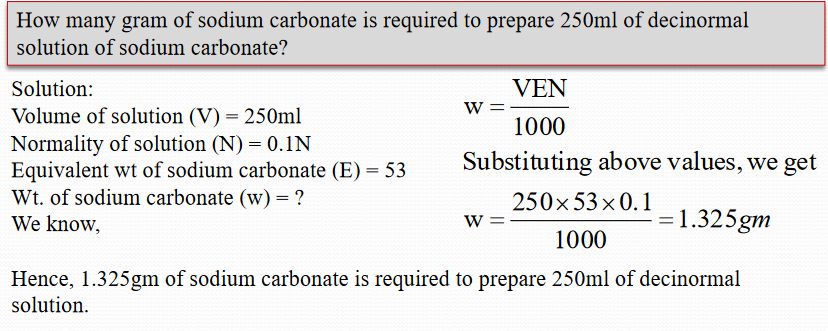### Calculate the concentration of solution obtained by dissolving 0.63gm of oxalic acid into 200ml of solution.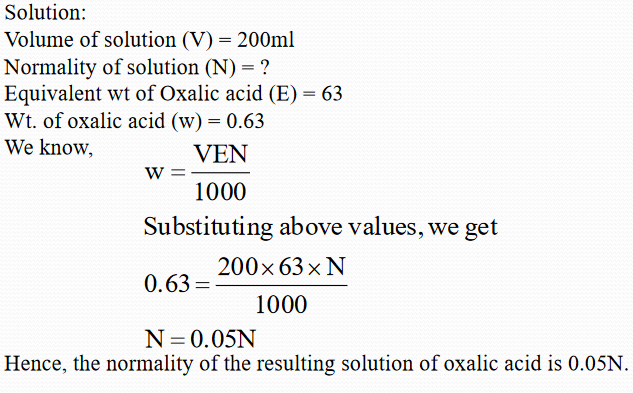### Molarity

Molarity of a solution may be defined as the no. of moles of solute present in one liter of
the solution. It is represented as M.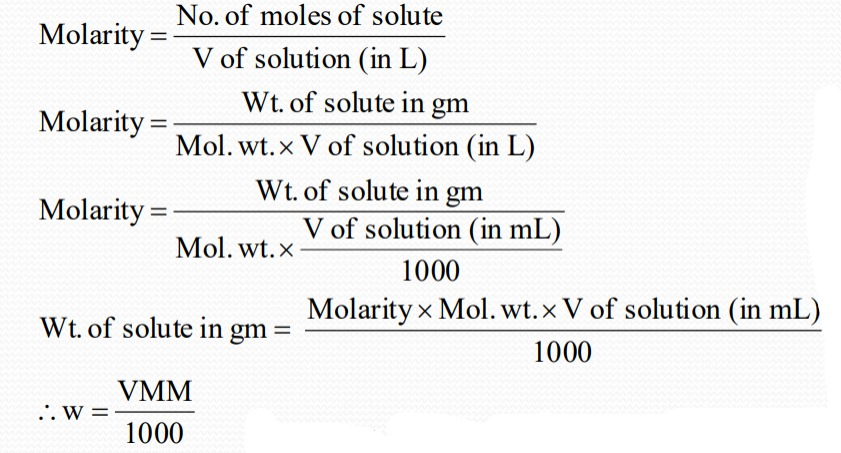The solution obtained by dissolving one gram equivalent weight of solute in one liter of solution is called normal solution.

No\ of\ Moles = \frac{Wt\ of\ Substance\ ( gm)}{Mol. \ Wt}

Similarly, the solution obtained by dissolving 1/10th mole of solute in one liter of solution is called decimolar solution.

### Gm/liter:

The amount of solute in gram that is present in one liter or 1000ml of solution is called
gram per liter.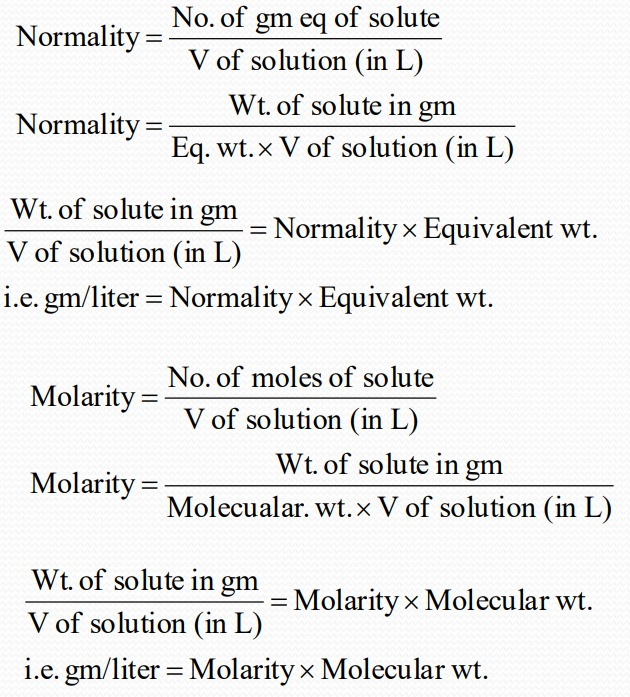### Relationship between normality and molarity: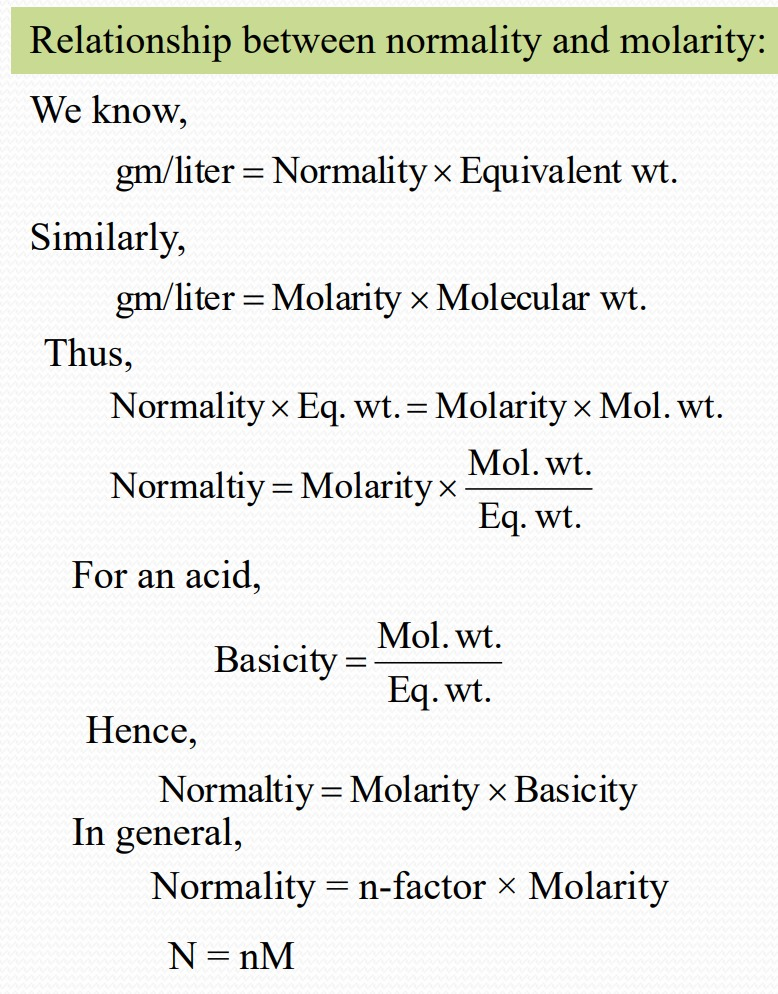### Percentage (%)

The amount of solute in gram that is present in 100ml of solution is called percentage (by volume).### Some Important Formula### 1) Express the following in g. eqvt. and moles

#### i) 1.06 g. Na 2 CO 3

We have, g. eqvt.

= frac{given wt}{eqvtwt}

= \frac{1.06}{53} = 0.02

no. of moles

= frac{given wt}{eqvtwt}

= \frac{1.06}{106} = 0.01

#### ii) 150 ml. of 0.1M NaOH solution

We have, g. eqvt. = normality x vol. in litre

= 0.1*\frac{150}{1000} (in NaOH, N =M)

= 0.015

No. of moles = molarity x vol.in litre

= 0.1*\frac{150}{1000}

= 0.015

### 2) Calculate the wt. of anhydrous Na2CO3 required to prepare 250 ml. of  \frac{N}{20}(f = 1.01) solution.

We have, X = \frac{NEV}{1000}

= \frac{1*1.01*53*250}{20*1000}

= 0.67 g

### 3) How many kg of wet NaOH containing 12% water are required to make 120 L of a 0.25 N solution.

We have,X = \frac{NEV}{1000}

= \frac{0.25*40*120*1000}{1000}

= 1200 g.=1.2 kg.

Let the wt. of wet NaOH be y g.

A/Q, (100-12) % of y = x

or, \frac{88}{100}*y=1.2 kg.

or, y = 1.363 kg.

### 4) You are given a L of semi molar solution of oxalic acid. What volume of water should be added into it to make it exactly decinormal?

Here, V1 = 1L         V2 = (1+x) L

S1 = \frac{M}{2} = 1N

S2 = \frac{N}{10} = 0.1 N

(In oxalic acid, N = 2M)

We have, from normality equation:

V1 S1 = V2 S2

or, 1 * 1 = (1 + x)  * \frac{N}{10}

or, 1+ x =10

or, x = 9 L

### 5) Concentrated hydrochloric acid has a specific gravity of 1.16 & contains 32% HCl by wt. calculate the vol. of this acid which would be required to make 10 L of a normal solution of the acid.

#### a) Calculation of concentration of conc. HCl

We have, Normality   = \frac{\% (\frac{w}{w})*sp.  gravity*10}{eqvt wt\ldotp }

= \frac{32*1.16*10}{36.5}

= 10.17 N

#### b) Calculation of vol.

Here, V1 =?     V 2 = 10 L

S1 = 10.17 N     S2 = 1 N

We have, from normality equation:

V_1 S_1 = V_2 S_2

or, V 1 x 10.17 N = 10 x 1 N

V1= 0.983 L

6) x g. of oxalic acid reacts completely with 20 ml. of \frac{N}{10}(f = 1.06)  KMnO_4 solution. Calculate the value of x.

Here, g. eqvt. of oxalic acid = g. eqvt. of KMnO4

or, (\frac{given wt.}{eq. wt})_Oxalic acid = ( normality*g. eqvt. of KMnO_4)

\frac{X}{63} = 0.1*1.06*\frac{20}{1000}

X = 63*0.1*1.06*0.02g.

X = 0.133g.

### 7) If 20 ml. of \frac{N}{20}(f = 2.06) H_2SO_4 and 30 ml.of \frac{N}{10}(f = 1.12) HNO 3 are mixed together, calculate the normality of mixture.

We have,  V_m S_m = V_1 S_1 + V_2 S_2

or, (20 +30) * S_m = 20 * 0.05 * 2.06 + 30 * 0.1 * 1.12

or, S_m x 50 = 2.06 + 3.36

or, S_m = 5.42/50

∴ S_m = 0.1084 N

### 8) What volume of semi normal and centimolar H_2SO_4 solutions should be mixed in order to prepare 1.5 L of decinormal solution of H_2SO_4?

Let, V_1 = y         V_2 = 1.5-y

S_1 = \frac{N}{2}

S_2 = \frac{M}{100} = \frac{N}{50}

We have,  V_m S_m = V_1 S_1 + V_2 S_2

or, 1.5 * \frac{N}{10} = y * \frac{N}{2} + (1.5-y) * \frac{N}{50}

or, 0.15 = 0.5y + 0.03 – 0.02y

or, 0.48y = 0.12

or, y = \frac{0.12}{0.48} = 0.25 L

Thus, the vol. of semi normal H_2SO_4 = y = 0.25 L

The vol. of centimolar H_2SO_4  = 1.5-y = 1.5-0.25=1.25 L

### 9) 30 c.c. of 0.2N HCl, 10 c.c. of 1N H_2SO_4 , 20 c.c. of \frac{N}{10}HNO3 and 40 c.c. of water are mixed together. What will be the normality of the mixture?

We have, V_m S_m = V_1 S_1 + V_2 S_2+V_3 S_3 + V_4 S_4

or, 100 x S_m = 30 x 0.2 + 10 x 1 +20 x 0.1 + 40 x 0

or, 100 x S_m = 6 + 10 + 2 + 0

or, S_m =  \frac{18}{100} = 0.18N

### Remaining Numericals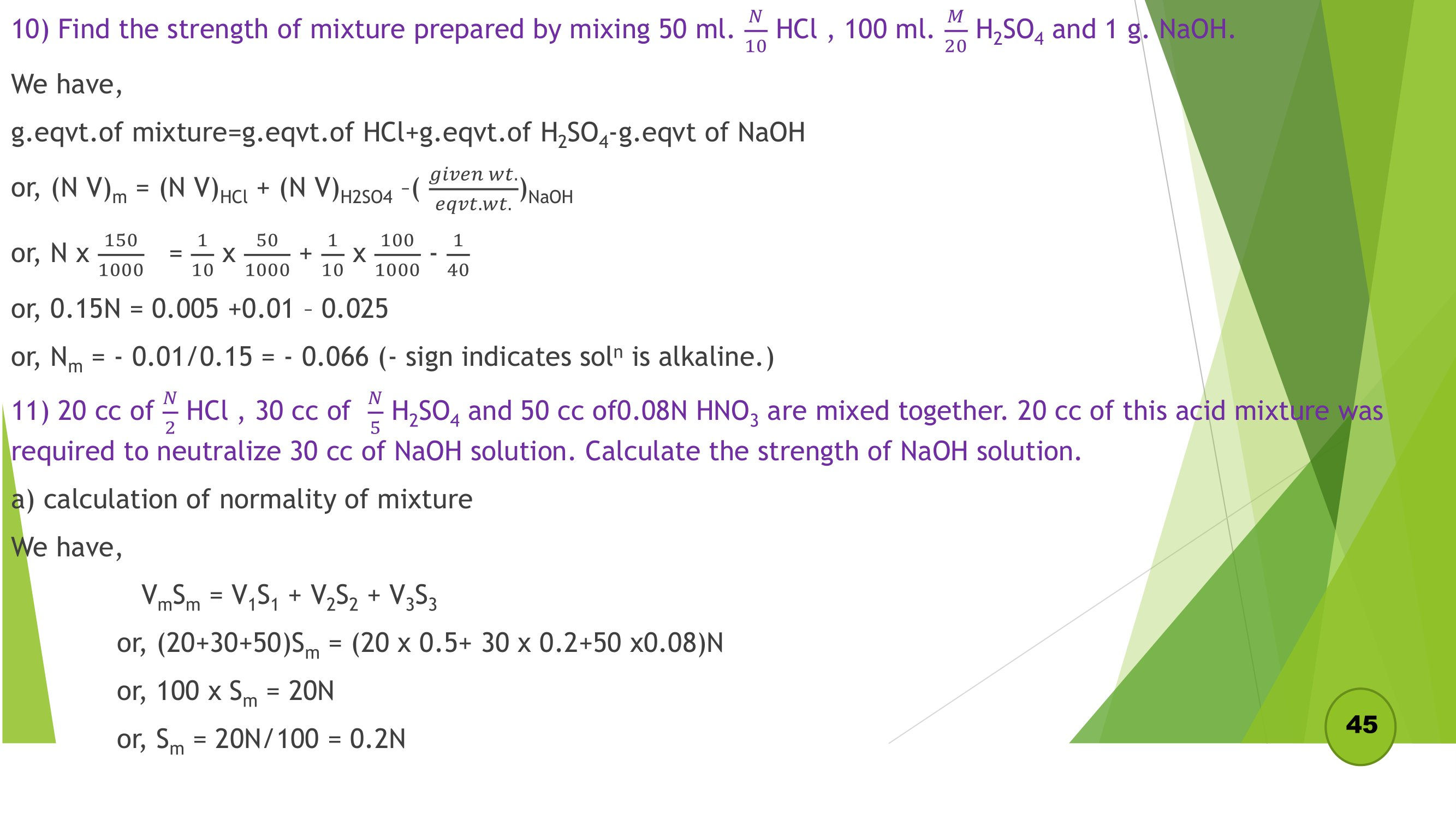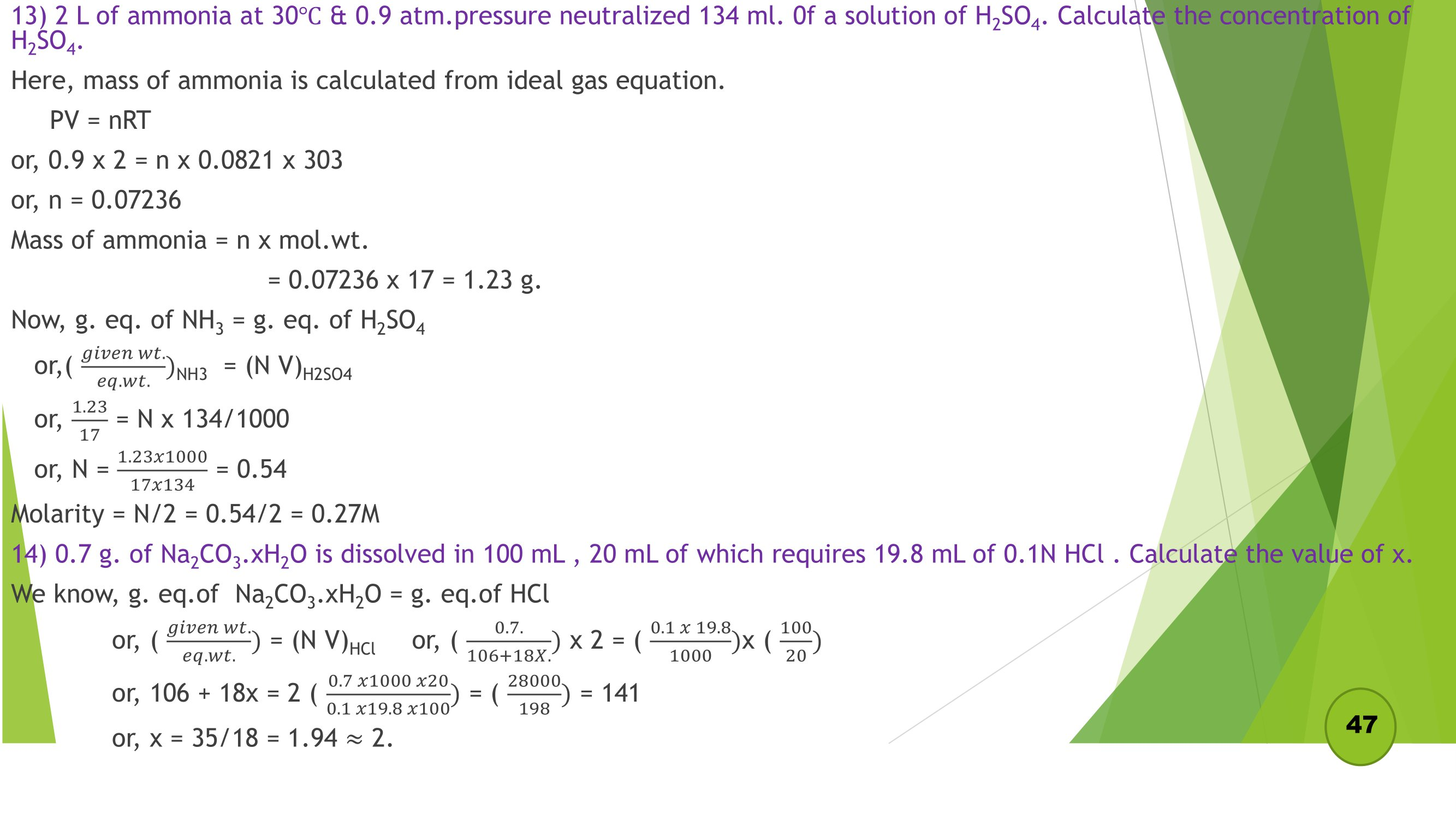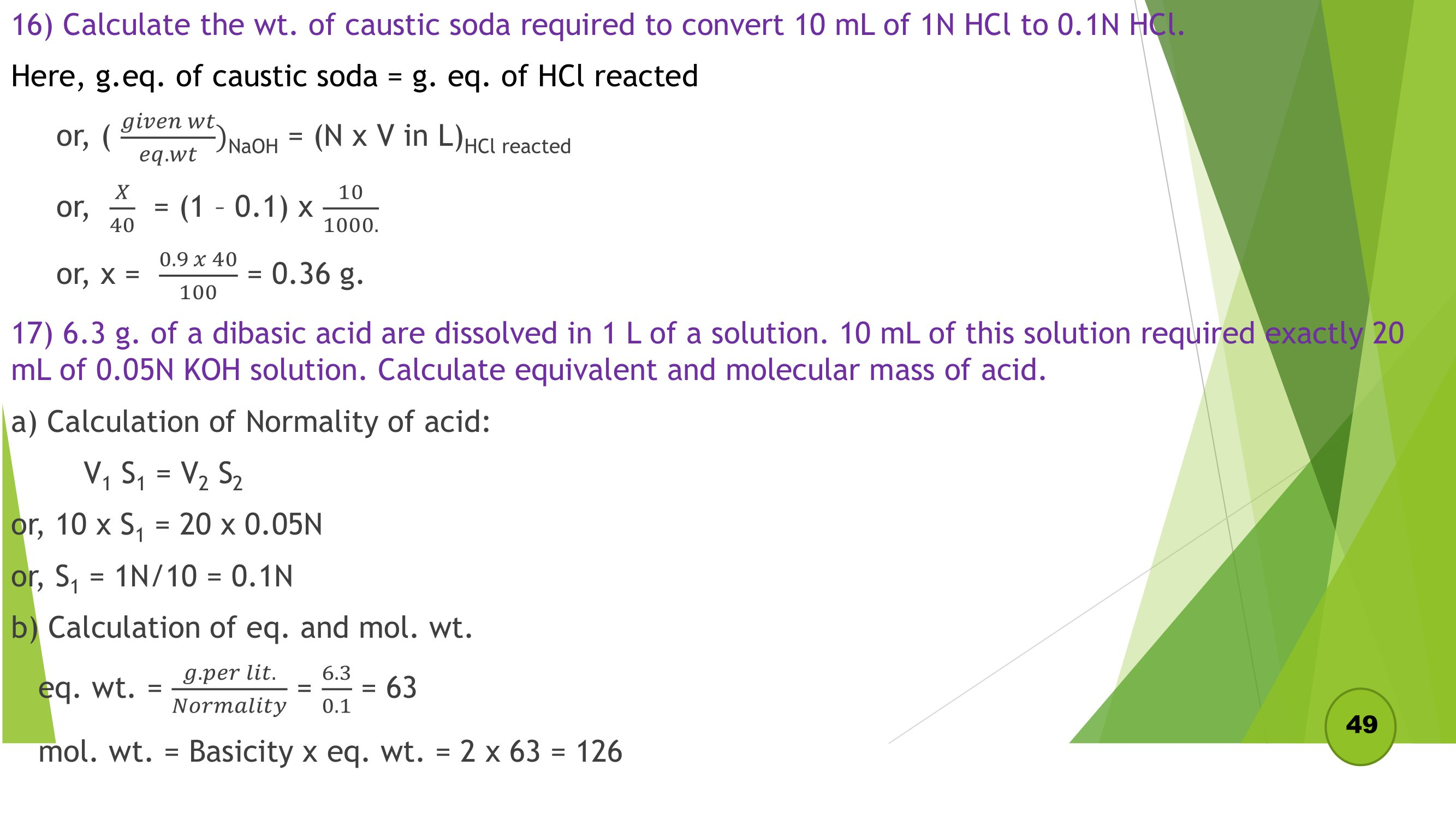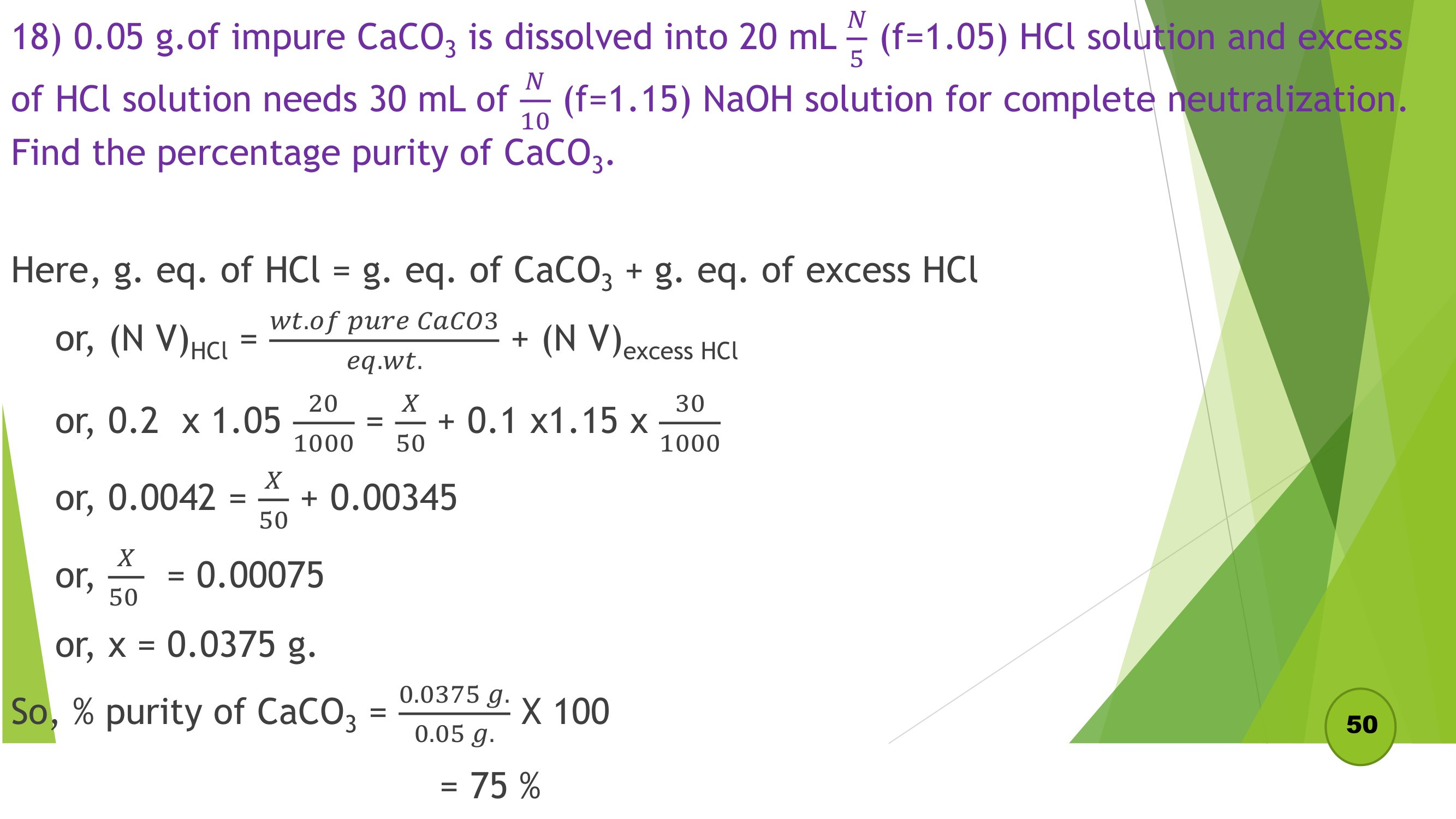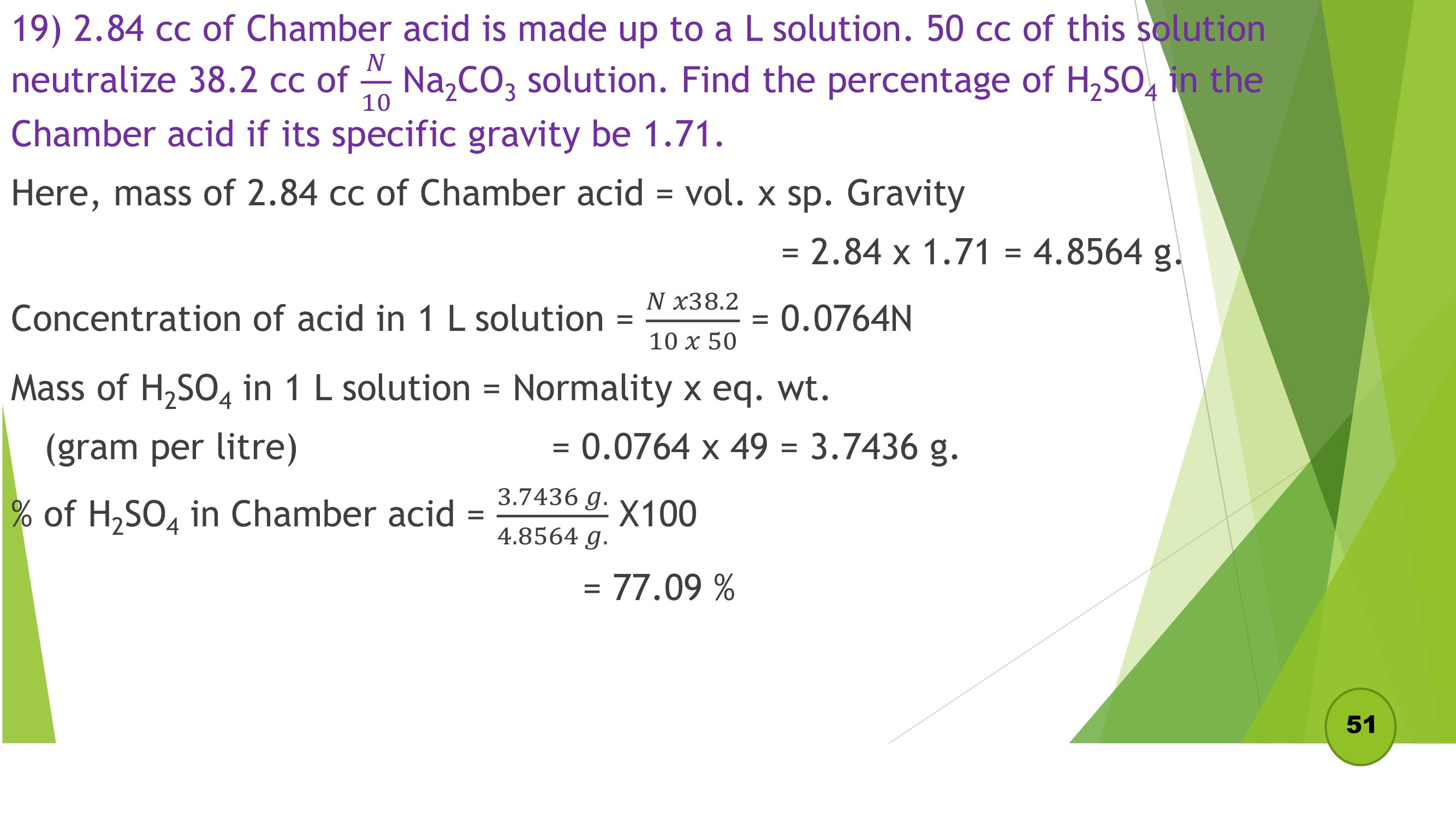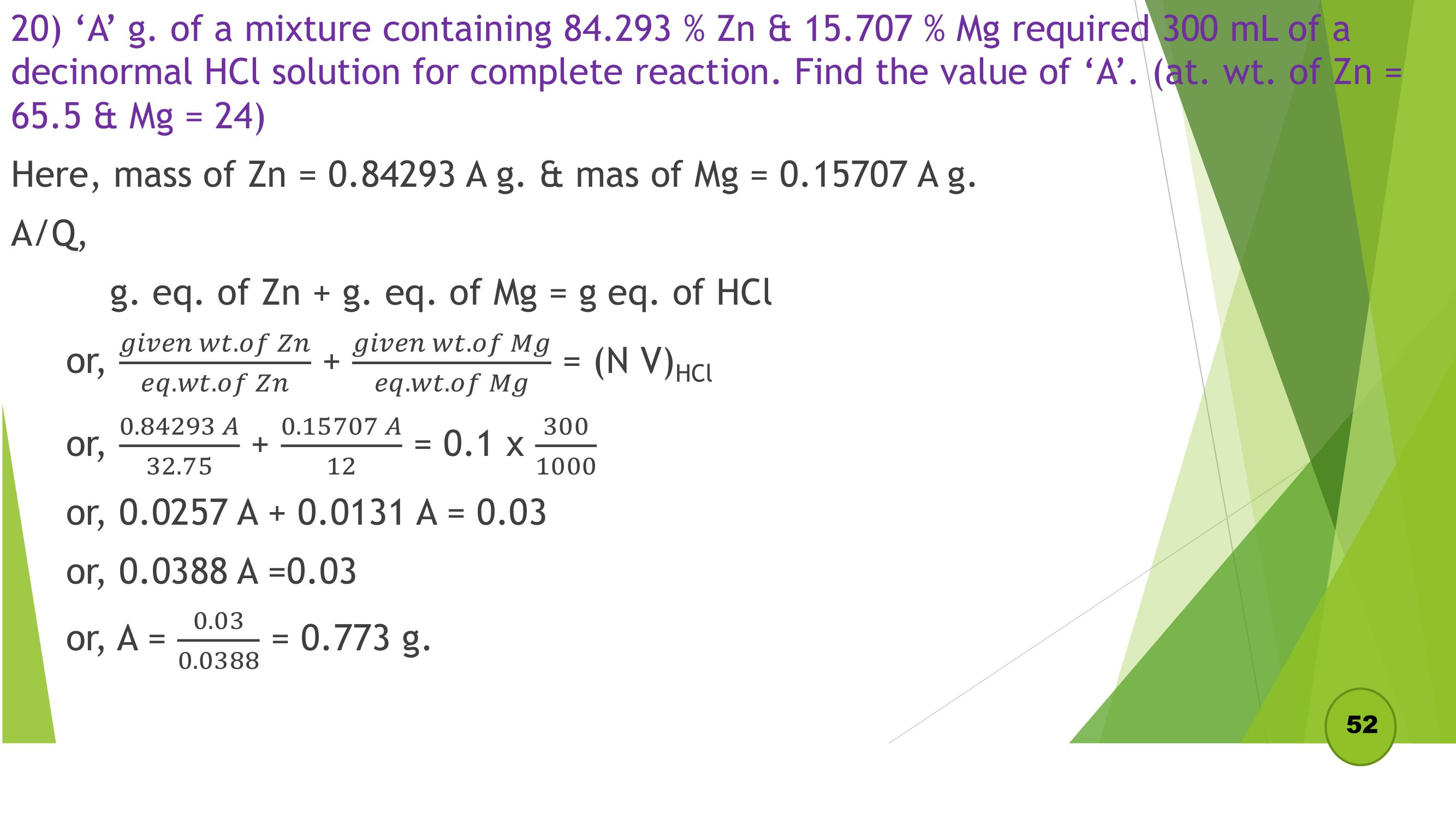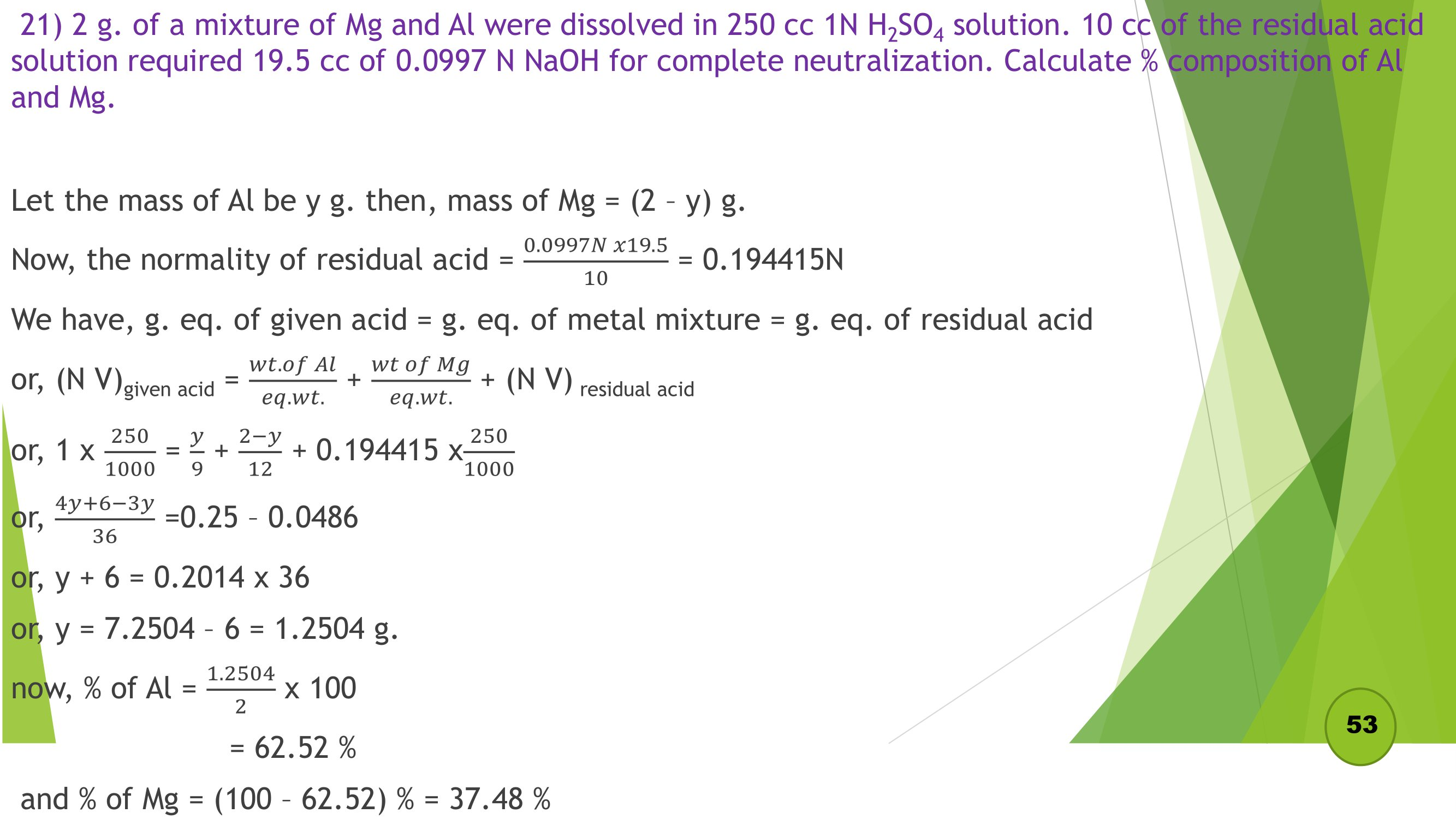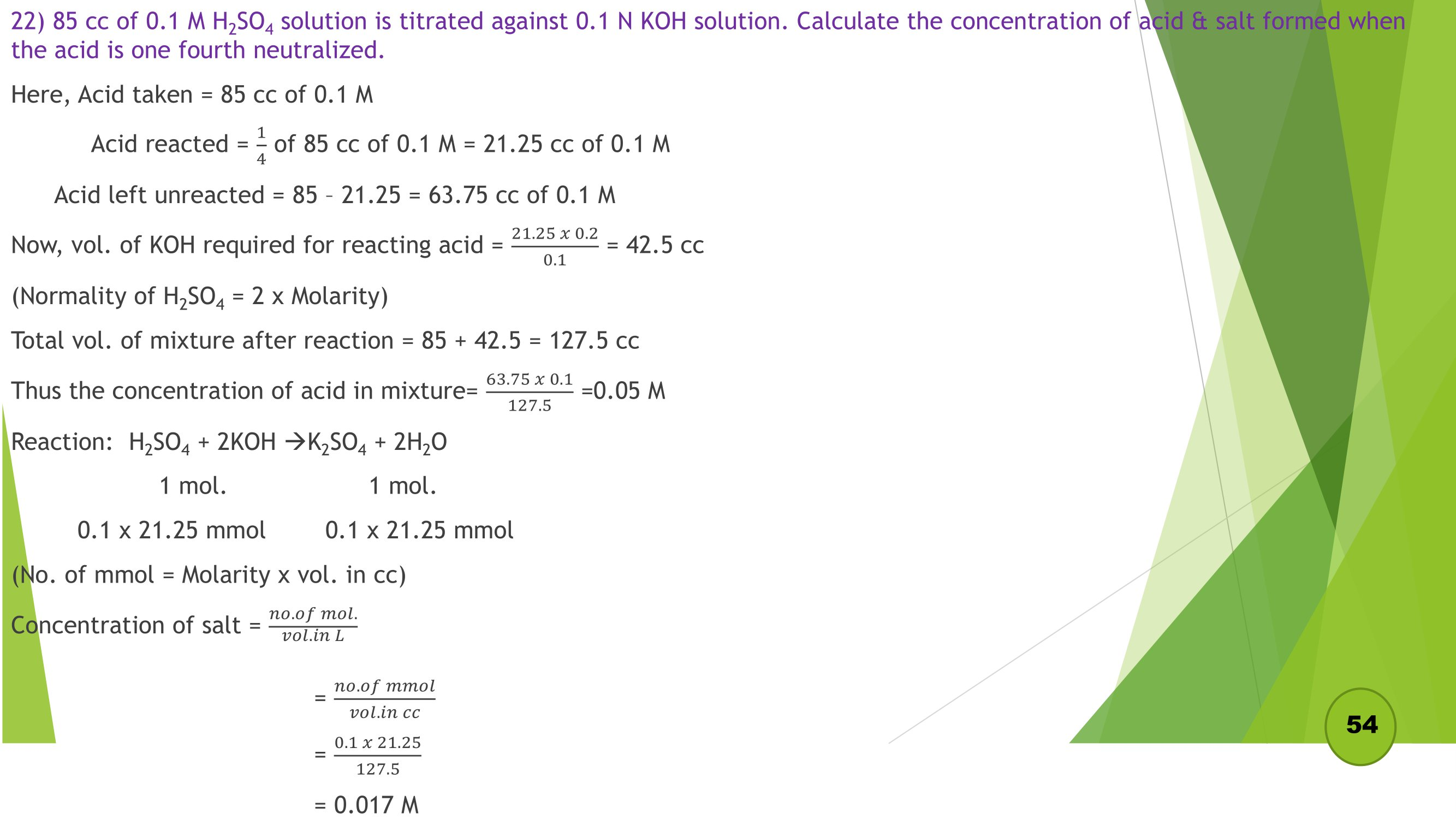## Volumetric Analysis Numericals PDF

Read: Volumetric Analysis Note - Class 12 Chemistry
Getting Info...